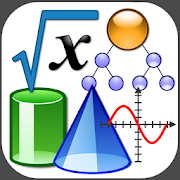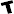Math TutorialsTeen
209
Mathematics tutorials of arithmetic, algebra, fractions, geometry, trigonometry...

From basic to advanced maths, learn watching tutorials.

You will learn basic operations like adding, substracting, multiplications...

And also advanced mathematics like functions, algebra, geometry...

Find in this app tutorials of:

- Arithmetic
- Algebra
- Trigonometry
- Fractions
- Discrete Math
- Statistics
- Limits
- Derivatives
- Physics

Find also math exercises to practice solving equations, fractions, basic operations...

All videos are played from youtube. We do not hold any videos in our servers. All credits and rights of the videos are for the channel owner.
Collapse

Review Policy
4.3
209 total
5
4
3
2
1

What's New

Math tutorials - Geometry - Algebra - Arithmetic
Discrete Math
Functions - Limits - Derivatives
Statistics
Added community and player exercise rank.
Added math books and Matrices exercises.
Added search engine and recently watched tab.
Added math podcasts and papers.
Collapse

Updated
October 2, 2019
Size
7.3M
Installs
50,000+
Current Version
1.59
Requires Android
4.2 and up
Content Rating
Interactive Elements
Users Interact
Permissions
Offered By
duhnnae
Developer
Calle 7 #1 este-58 Torre 12 Apto 502 Chía - Cundinamarca